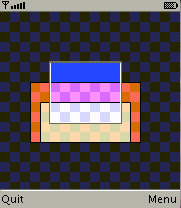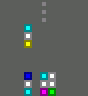-=+=- -=+=- -=+=- -=+=- -=+=- -=+=- -=+=- -=+=- -=+=- -=+=- -=+=- -=+=- -=+=- -=+=- -=+=- -=+=- -=+=- -=+=- -=+=- -=+=- -=+=- -=+=- -=+=- -=+=- -=+=- -=+=- -=+=- -=+=- -=+=- -=+=- (c) WidthPadding Industries 1987 0|92|0 -=+=- -=+=- -=+=- -=+=- -=+=- -=+=- -=+=- -=+=- -=+=- -=+=- -=+=- -=+=- -=+=- -=+=- -=+=- -=+=- -=+=- -=+=- -=+=- -=+=- -=+=- -=+=- -=+=- -=+=- -=+=- -=+=- -=+=- -=+=- -=+=- -=+=-### Pio

View Profile, Send Private Message
Recent Posts, Showcases, Articles, Code Snippets, Links, Wed.Workshop, Blog

### Latest Blog Entries

Pio# MIDlet Pascal and touch screen

17th April 2009

here are my latest discoveries

Pio# Swarming

29th September 2008

for two players

### Latest Showcases

Pio# POCA (reboot)

26th December 2020Remove all arrows without geting hit

Pio# SlotM

27th July 2018A simple simulation of slot machine.

Pio13th April 2015Game and watch

Pio# Jump

2nd March 2010One button rope skipping

Pio# Entangled rectangles mobile

12th April 2009Overlap the rectangles

Pio# Generic Block Game

16th September 2008Configurable falling blocks game